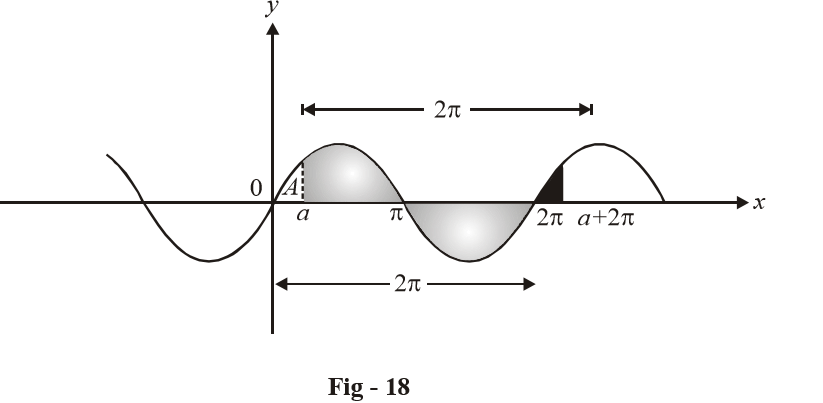# Integrating a Periodic Function

(13) If $$f(x)$$ is a periodic function with period T, then the area under $$f(x)$$ for n periods would be n times the area under $$f(x)$$ for one period, i.e.

$\int\limits_0^{nT} {f(x)\,dx = n\int\limits_0^T {f(x)\,dx} }$

Now, consider the periodic function $$f(x) = \sin x$$ as an example. The period of $$\sin x\,\,{\rm{is }}2\pi$$ .Suppose we intend to calculate $$\int\limits_a^{a + 2\pi } {\sin x\,dx}$$ as depicted above. Notice that the darkly shaded area in the interval $$[2\pi ,\,\,a + 2\pi ]$$ can precisely cover the area marked as A. Thus,

$\int\limits_a^{a + 2\pi } {\sin x\,dx} = \int\limits_0^{2\pi } {\sin x\,\,dx}$

This will hold true for every periodic function, i.e.

$$\int\limits_a^{a + T} {f(x)\,dx} = \int\limits_0^T {f(x)\,dx}$$ (where T is the period of f (x))

This also implies that

\begin{align}& \quad\qquad\;\int\limits_{a}^{a+nT}{f(x)dx=\int\limits_{0}^{nT}{f(x)dx=n}\int\limits_{0}^{T}{f(x)dx}} \\ & \text{and}\quad \int\limits_{a+nT}^{b+nT}{f(x)dx=\int\limits_{a}^{b}{f(x)dx}} \\ & \text{and}\quad\text{ }\!\!~\!\!\text{ }\int\limits_{a}^{b+nT}{f(x)dx=\int\limits_{a}^{b}{f(x)dx+n\int\limits_{0}^{T}{f(x)dx}}}\text{ } \\ \end{align}

Example –18

Show that $$\int\limits_0^{n\pi + V} {|\sin x|dx = (2n + 1) - \cos V,}$$ where n is a positive integer and $$V=[0,\pi )$$

Solution: $$f(x) = |\sin x|$$ is periodic with period p .

Therefore, as described in property-13,

\begin{align}&\int\limits_0^{n\pi + V} {|\sin x|dx} = \int\limits_0^V {|\sin x|dx + n\int\limits_0^\pi {|\sin x|dx} } \\ \,\,\,\,\,\,\,\,\,\,\,\,\,\,\,\,\,\,\,\,\,\,\,\,\,\,\,\,\, &\qquad\qquad\qquad= \int\limits_0^V {\sin xdx} + n\int\limits_0^\pi {\sin xdx} & & & \left[ \begin{gathered}\because \ \,\,\,\sin x \ge 0 \\\,\,\,\,\,\,{\text{for}}\,x \in [0,\pi ) \\\end{gathered} \right] \hfill \\ \,\,\,\,\,\,\,\,\,\,\,\,\,\,\,\,\,\,\,\,\,\,\,\,\,\,\,\, &\qquad\qquad\qquad= \left. { - \cos x} \right|_0^V + \left. {n( - \cos x)} \right|_0^\pi\\ \\ \,\,\,\,\,\,\,\,\,\,\,\,\,\,\,\,\,\,\,\,\,\,\,\,\,\,\,\, &\qquad\qquad\qquad= 1 - \cos V + n(2)\\ \\\,\,\,\,\,\,\,\,\,\,\,\,\,\,\,\,\,\,\,\,\,\,\,\,\,\,\,\, &\qquad\qquad\qquad= (2n + 1) - \cos V \\\end{align}

Example –19

Evaluate $$\int\limits_0^{n\pi } {\sqrt {1 - \cos 2x} \,dx,}$$ where n is a positive integer.

Solution:

$\begin{array}{l}I = \int\limits_0^{n\pi } {\sqrt {1 - \cos 2x} \,dx} \\\,\,\,\, = \int\limits_0^{n\pi } {\sqrt {2{{\sin }^2}x} \,dx} \,\,\,\,\\\,\,\, = \sqrt 2 \int\limits_0^{n\pi } {|\sin x|dx} \\\,\,\,\, = \sqrt 2 n\int\limits_0^\pi {|\sin x|dx} & & & ({\rm{Property - }}13)\\\,\,\,\, = 2\sqrt 2 n\end{array}$